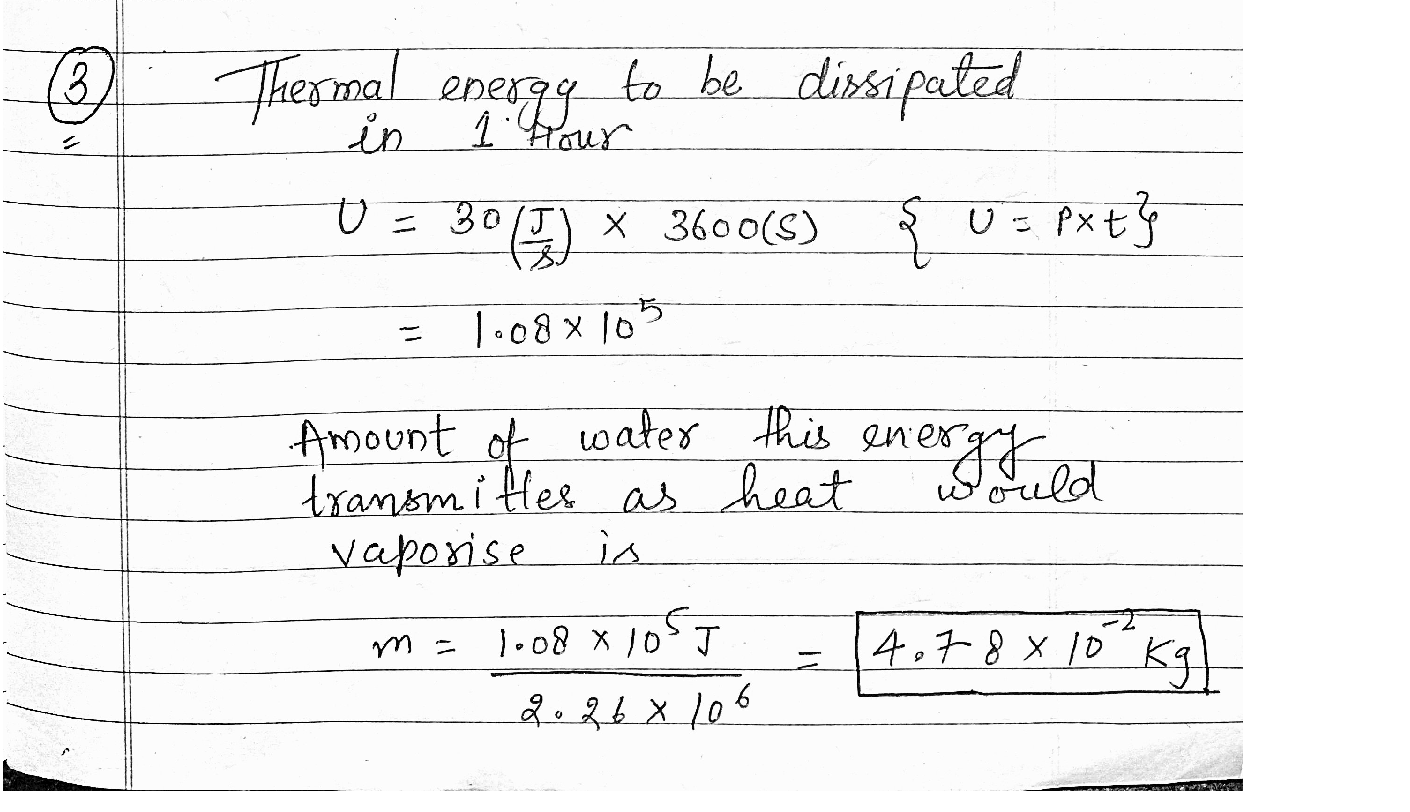Question

Modern Physics

3. During the game, the metabolism of(10)basketball players often increases by as much as 30.0 W. How much perspiration must a player vaporize per hour to dissipate this extra thermal energy?(You might want to review the concepts

of power and heat of vaporization from Physics 11. Also, assume that perspiration is simply pure water.)

Power is covered on page 202 of the text and is measured in units or J/s or watts.

Assume perspiration starts at a temperature of 37°C.*

See pages 247-248 of the text to help calculate the amount of heat needed to raise the temperature of a substance. Specific heat capacity of water is given on page 248; use Q = mc AT formula when calculating heat energy needed to raise water temperature to 100°C.

See pages 252-254 of the text to calculate heat needed to completely boil water at 100°C. The heat of vapourization of water is given on page254 of the text; use Q = mH, formula to calculate amount of heat energy needed to completely vapourize the perspiration.

D) 4. Figure 2.24 shows two identical billiard balls before and after a glancing collision. Determine the speed of the other ball.Verified### Question 52422Modern Physics

1A cruiser moves away from the planet Hoth at a speed of 0.5c. A frigate follows, catching up at a speed of 0.25c as viewed from the cruiser. With what speed does the frigate appear to be moving according to observers on the planet? You should use the relativistic velocity transformation, clearly defining all the parameters used.
u^{\prime}=\frac{u-v}{1-\frac{u v}{c^{2}}}

### Question 44658Modern Physics

Question 5: A spaceman goes out on a trip. First, he travels at 1/3 the speed of light in one direction. Then he comes back home at 2/3 of the speed of light. On Earth 9 years have passed,by how much has the spaceman aged? (Make sure you draw a space-time diagram).

### Question 44657Modern Physics

Question 4: The typical pole for pole vaulting is 17 feet long. How fast would a pole vaulter have to run (in the direction of the pole) in order for a spectator sitting in the stands to see it 15feet long?

### Question 44656Modern Physics

Question 3: If you weigh 150 lb in Chicago, how much do you weigh on top of mount Everest?(mount Everest is 8,848 m high).

### Question 44655Modern Physics

Question 2: As a result of an explosion a stationary object breaks into three pieces. One piece flies east, one west and one north.
Explain why this is impossible.
Now, there are only two pieces; one flies east the other west. One piece weighs three times as much as the other. What is the ratio of the kinetic energies of the two pieces?

### Question 44654Modern Physics

Question 1: The international Space Station (ISS) orbits the Earth once every 90 mins at an-altitude of 409 km. How high would it have to be in order to be to be in geosynchronous orbit?(make sure you show how you worked it out).

### Question 44179Modern Physics

Question 6: A "star" made of ideal gas radiates away 10% of its energy while remaining in hydrostatic balance. Assuming that there are no nuclear reactions
Does the radius of the "star" increases or decreases? By how much?
Does the (average) temperature of the "star" increases or decreases? By how much?

### Question 44178Modern Physics

5: There are two stars, one has twice the luminosity of the other and half the radius.
What is the ratio of their temperatures?
What is the ratio of their (apparent) brightness if the more luminous one is 100 times more distant than the other.

### Question 44177Modern Physics

Question 4: According to general relativity people who live in hi-rise buildings age at differentrates depending on their floor.
Is that true?
Which floor ages fastest?

### Question 44176Modern Physics

Question 3: Consider two time-like events P and Q. P is at the space-time origin P=(0,0); Q is at the space origin but one second later Q=(0,1).
Show that the order of events is preserved by all observers travelling at less than the speed of light.
Now events P and Q are space-like. P is still at the origin, but Q is simultaneous with P but one meter to the right 0=(1.0)
.Show that the order of events is not preserved by observers travelling at less than the speed of light.

### Submit query